# Calculate the final concentration of the solution when water is added to prepare each of the...

Calculate the final concentration of the solution when water is added to prepare each of the following solutions. 22.0 mL of a 10.0 %(m/v) K2SO4 solution is diluted to 60.0 mL & 80.0 mL of a 7.60 M NaOH solution is diluted to 245 mL

(a). Solution.1 :- 22.0 mL of a 10.0 %(m/v) K2SO4 solution is diluted to 60.0 mL :-

10.0%(m/v) of K2SO4 means, 10.0 g of K2SO4 are present in 100.0 mL of solution

Therefore,

22.0 mL of solution contains = 10.0 g x 22.0 mL / 100.0 mL = 2.2 g of K2SO4

Number of moles of K2SO4 = Mass of K2SO4 / Gram molar mass of K2SO4

= 2.2 g / 174.259 g/mol

= 0.01262 mol of K2SO4

Total volume becomes = 22.0 mL + 60.0 mL = 82.0 mL = 0.082 L

Now,

Final concentration of K2SO4 = Number of moles of K2SO4 / Volume in L

= 0.01262 mol / 0.082 L

= 0.154 M

 Therefore, final concentration of K2SO4 = 0.154 M

(b). Solution.2 :- 80.0 mL of a 7.60 M NaOH solution is diluted to 245 mL :-

Number of moles of NaOH = Molarity of NaOH x Volume in L

= 7.60 M x 0.080 L

= 0.608 mol

Total volume becomes = 80.0 mL + 245 mL = 325 mL = 0.325 L

Now,

Final concentration of NaOH= Number of moles of NaOH / Volume in L

= 0.608 mol / 0.325 L

=1.87 M

 Therefore, final concentration of NaOH = 1.87 M

#### Earn Coin

Coins can be redeemed for fabulous gifts.

Similar Homework Help Questions
• ### Calculate the final concentration of each of the following diluted solutions

Calculate the final concentration of each of the following diluted solutions.a.)A 10.0 mL sample of 15 % (m/v) KOH solution is diluted with water so that the final volume is 100.0 mL.b.)A 50.0 mL sample of 18 % (m/v) H2SO4 solution is added to water to give a final volume of 250 mL.

• ### Four SFS Thre Concentr decreas When the final volu (concentration factor volume (V) as predic STUDY...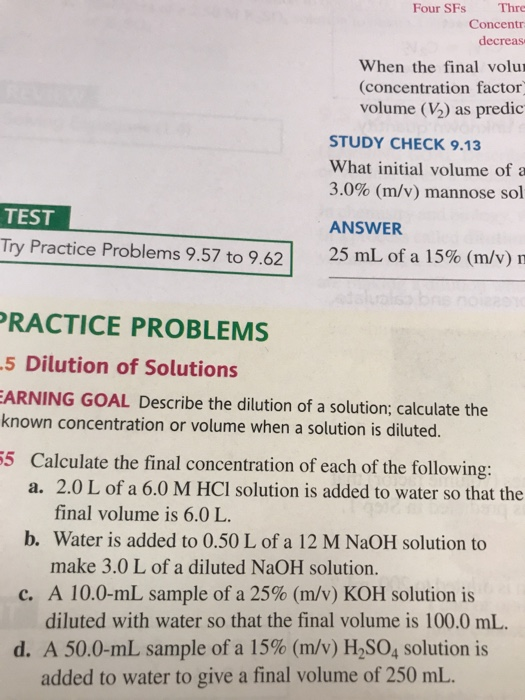Four SFS Thre Concentr decreas When the final volu (concentration factor volume (V) as predic STUDY CHECK 9.13 What initial volume of a 3.0% (m/v) mannose sol TEST Try Practice Problems 9.57 to 9.62|| ANSWER 25 mL of a 15% (m/v) PRACTICE PROBLEMS -5 Dilution of Solutions EARNING GOAL Describe the dilution of a solution; calculate the known concentration or volume when a solution is diluted. 55 Calculate the final concentration of each of the following: a. 2.0 L of...

• ### a. What is the new % (m/v) concentration when water is added to 25.0 mL of...

a. What is the new % (m/v) concentration when water is added to 25.0 mL of 20.0% (m/v) NaOH to make 300.0 mL of a diluted NaOH solution? b. What is the new molarity of the solution when water is added to 125.0 mL of 2.50 M NaOH to make 300. mL of a diluted NaOH solution?

• ### Calculate the volume, in milliliters, for each of the following that provides the given amount of...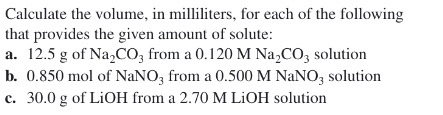Calculate the volume, in milliliters, for each of the following that provides the given amount of solute: a. 12.5 g of Na2CO, from a 0.120 M Na CO, solution b. 0.850 mol of NaNO, from a 0.500 M NaNO, solution c. 30.0 g of LiOH from a 2.70 M LiOH solution Calculate the final concentration of each of the following: a. 2.0 L of a 6.0 M HCl solution is added to water so that the final volume is 6.0...

• ### Calculate the final concentration of each of the following diluted solutions. Part A A 50.6 mL...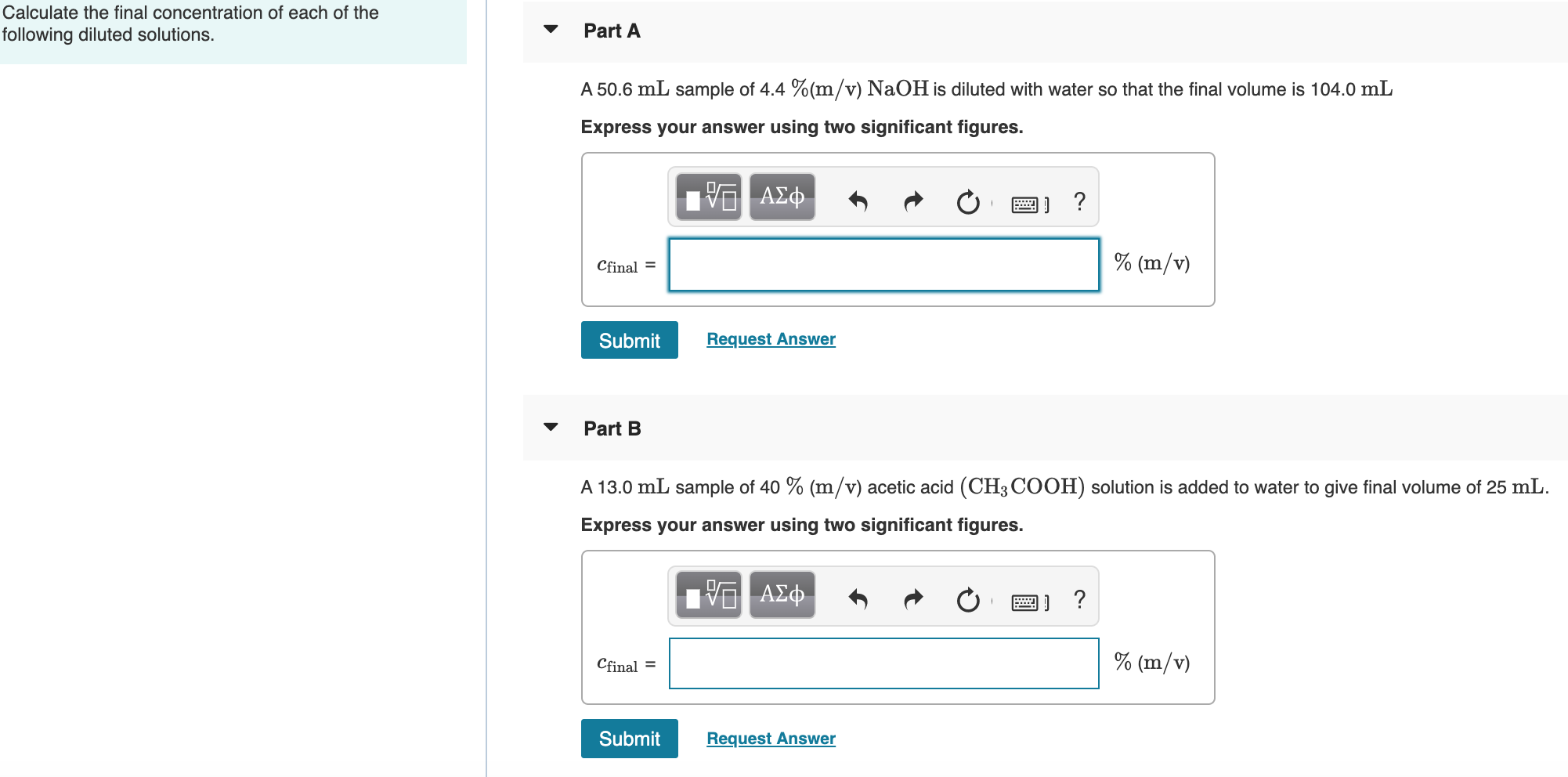Calculate the final concentration of each of the following diluted solutions. Part A A 50.6 mL sample of 4. aOH is diluted with water so that the final volume is 104.0 mL Express your answer using two significant figures. PO AQ R O 2 ? Chimal = Cfinal = % (m/v) Submit Request Answer Part B A 13.0 mL sample of 40 % (m/v) acetic acid (CH3 COOH) solution is added to water to give final volume of 25 mL....

• ### 1. Calculate the molarity of the following solutions: a. 318 g Mg Br, in 859 ml...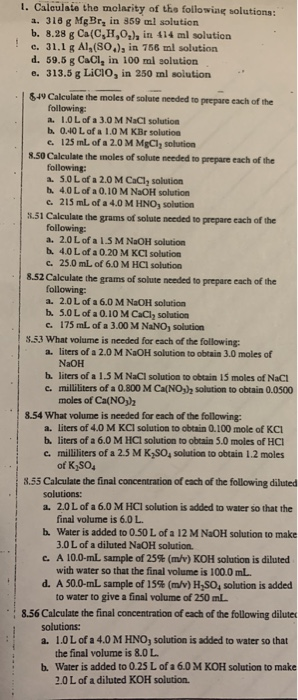1. Calculate the molarity of the following solutions: a. 318 g Mg Br, in 859 ml solution b. 8.28 g Ca(C,H,O,), in 414 ml solution c. 31,1 g Al,(80.), in 766 ml solution d. 59.8 g CaCl, in 100 ml solution e. 313.5 g LICIO, in 250 ml solution | 849 Calculate the moles of solute needed to prepare each of the following: 2. LOL of a 3.0 M NaCl solution b. 0.40 L of a 1.0 M KBr solution...

• ### When water is added to solids to prepare a solution, the volume of the solution is...

When water is added to solids to prepare a solution, the volume of the solution is not necessarily the same as the volume of the water added. For dilute solutions the two volumes are very similar. Assuming the volume of solutions prepared is 10.0 mL calculate the following based on the mass 0.2610 of ammonium sulfate. 1. The molarity of ammonium sulfate in the solution expressed to 3 decimal places. 2. the molarity of ammonium ions in the solution 3....

• ### A 10.0 mL sample of a 25% (m/v) KOH solution is diluted with water so that the final volume is 100.0 mL. Calculate the f...

A 10.0 mL sample of a 25% (m/v) KOH solution is diluted with water so that the final volume is 100.0 mL. Calculate the final concentration.

• ### a) Calculate the final concentration when 25.0 mL of an 18.0 M HCl solution is diluted...

a) Calculate the final concentration when 25.0 mL of an 18.0 M HCl solution is diluted to 0.500 L. b) Calculate the volume required when an 8.50 M KOH solution is diluted to form 95.0 mL of a 0.500 M KOH solution

• ### 8-67 For each of the following solutions, how many milliliters of water should be added to...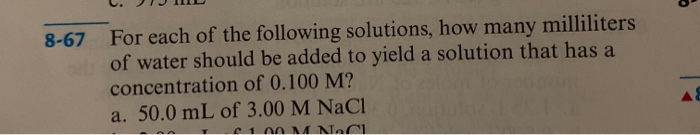8-67 For each of the following solutions, how many milliliters of water should be added to yield a solution that has a concentration of 0.100 M? a. 50.0 mL of 3.00 M NaCl 1 MANAC1 20.1J0 11 18103 8-69 Determine the final concentration of each of the following solutions after 20.0 mL of water has been added. a. 30.0 mL of 5.0 M NaCl solution b. 30.0 mL of 5.0 M AgNO, solution c. 30.0 mL of 7.5 M NaCl...

Free Homework Help App